# Configuring a Noise Analysis in Multisim

Publish Date: Jun 13, 2013 | 0 Ratings | 0.00 out of 5 | Print | Submit your review

## Overview

Multisim features a comprehensive suite of SPICE analyses for examining circuit behavior. These analyses range from the basic to sophisticated. Each analysis helps you to obtain valuable information such as the effects of component tolerances and sensitivities. For each analysis you need to set settings that will inform Multisim exactly what to analyze, and how.

Multisim simplifies the procedure for an advanced analysis by providing a configuration window. This abstracts away the complexities associated with SPICE syntax and configuration of an analysis. With this window you merely need to specify the parameter values and output nodes of interest.

This tutorial is part of the National Instruments SPICE Analysis Fundamentals Series. Each tutorial in this series provides you with step-by-step instructions on how to configure and run the different SPICE analyses available in Multisim.powerful simulation and analysis while abstracting the complexity of SPICE syntax.

### 1. Introduction

Noise is electrical or electromagnetic energy that reduces the quality of a signal. Noise affects digital, analog and all communications systems. Noise Analysis calculates the noise contribution from each resistor and semiconductor device at the specified output node. Multisim creates a noise model of the circuit using noise models of each resistor and semiconductor devices and then performs AC-like analysis. It calculates the noise contribution of each component and propagates it to the output of the circuit sweeping through the frequency range specified.

Multisim can model three different kinds of noise:

• Thermal noise (also known as Johnson, or white noise) is temperature dependent and caused by the thermal interaction between free electrons and vibrating ions in a conductor. Its frequency content is spread equally throughout the spectrum.
• Shot noise is caused by the discrete-particle nature of the current carriers in all forms of semiconductors. It is the major cause of transistor noise.
• Flicker noise is usually generated by BJTs and FETs and occurs in frequencies below 1 KHz. This is type of noise is also known as excess noise or pink noise. It is inversely proportional to frequency and directly proportional to temperature and DC current levels.

Multisim performs Noise Analysis using the following approach:

1. Each resistor and semiconductor device is considered a noise generator.
2. Each noise generator’s contribution is calculated and propagated by the appropriate transfer function to the output of the circuit.
3. The total output noise at the output node is the RMS (Root Mean Square) sum of the individual noise contribution.
4. The result is then divided by the gain from input source to the output source to get the equivalent input noise. This is the amount of noise which, if injected at the input source into a noiseless circuit, would cause the previously calculated amount of noise at the output.

### 2. Running Noise Analysis

Figure 1  shows a basic operational amplifier with a gain of 5.  You will use Noise Analysis to obtain results for noise voltage for R1 and R2 and display a graph of the noise spectrum across a frequency range between 1 Hz and 10 GHz.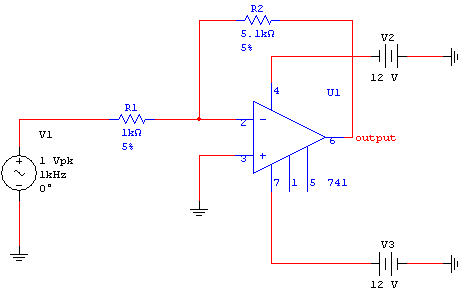Figure 1. Inverting amplifier circuit.

Complete the following steps to configure and run a Noise Analysis:

2. Select Simulate»Analyses»Noise Analysis. The Noise Analysis window opens. Table 1 describes the Analysis Parameters tab in detail.

Table 1. Parameters used in Noise Analysis.

 Parameter Meaning Input noise reference source Specifies the name of the independent voltage or current source that is to be the input reference source to which equivalent input noise is referred. Output node Specifies the node at which all noise contributions will be summed. Reference node Specifies the reference node for the output noise voltage. Change Filter Displays nodes contained within subcircuits or hierarchical blocks. There are three options: Display internal nodes. Displays nodes within hierarchical blocks and subcircuits. Display submodules. Displays components within semiconductor devices determined by the SPICE model of the semiconductor device. Display open pins. Displays all unconnected nodes of the circuit. Calculate power spectral density curves Generates a graph of the power spectral density. Points per summary Specifies how often the noise contributions of each noise generating device are reported. The recommended value is 1. Calculate total noise values Generates a table with total noise data.

Note: In SPICE, the command that performs a Noise Analysis has the following general form (the complete statement is more complex):

.NOISE <OUTPUT_VOLTAGE> <INPUT_SOURCE> <OUTPUT_INTERVAL>

Note that these parameters are similar to those defined in Table 1, however, in Multisim you do not have to worry about the SPICE syntax.

1. Configure the Analysis Parameters tab as shown in the following figure: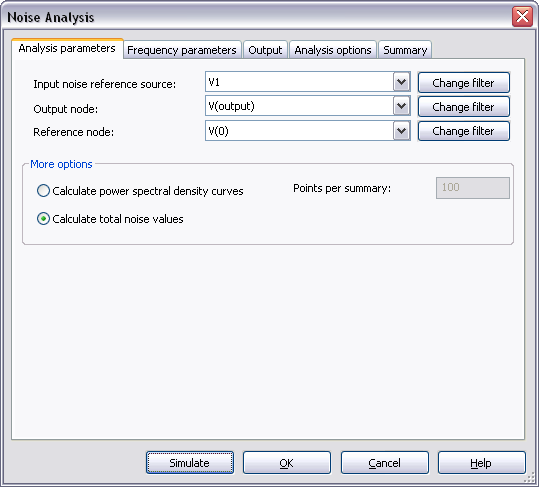Figure 2. Analysis parameters.

1. Select the Frequency Parameters tab. Leave the default settings as shown in Figure 3 (these settings are appropriate for most cases).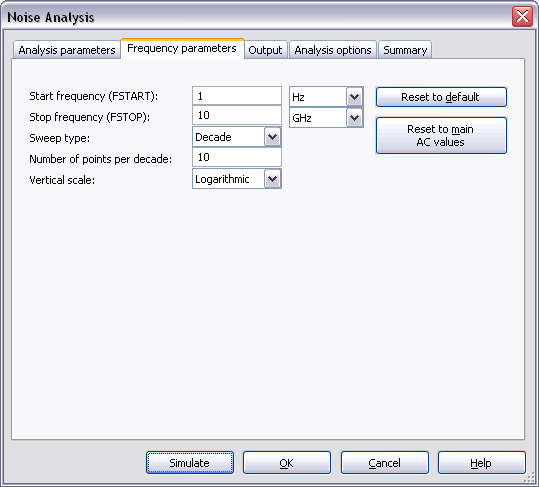Figure 3. Frequency parameters.

You can reset all the parameters to their default values by clicking the Reset to default button. To copy the settings from the current AC Analysis to this analysis, click Reset to main AC values.

Refer to the AC Analysis tutorial for more details on how to configure the Frequency Parameters tab.

1. Select the Output tab.
2. Select the Variables in circuit list, select All variables from the drop-down list, and then highlight inoise_total_rr1 from the list.
3. Click the Add button to move the variable to the right side under Selected variables for analysis.
4. Repeat this process for the inoise_total_rr2 variable. The Output tab will look as shown in Figure 4.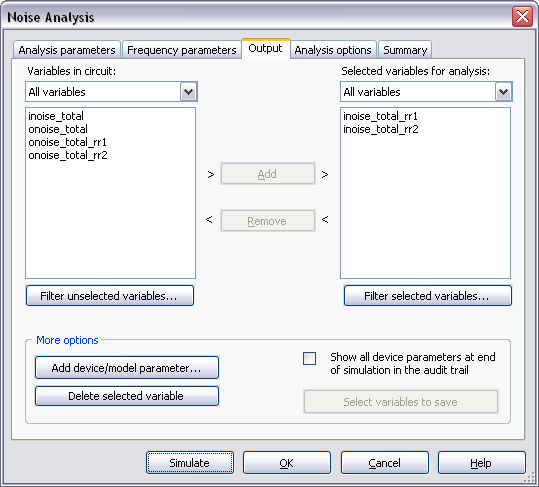Figure 4. Output variables for the Noise Analysis.

1. Click Simulate. The Grapher View opens and displays the noise contribution for each resistor (Figure 5).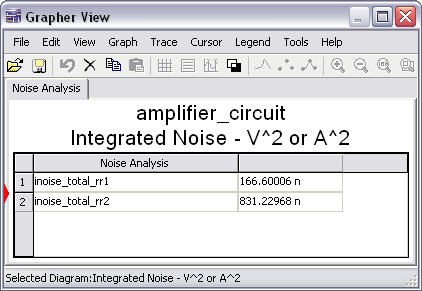Figure 5. Noise Analysis results.

In the next steps you will plot the power spectral density.

1. Close the Grapher View.
2. Select Simulate»Analyses»Noise Analysis. In the Analysis Parameters tab enable Calculate power spectral density curves.
3. Enter 1 in the Points per summary field.
4. Select the Output tab.
5. Add the variables onoise_rr1 and onoise_ rr2 to the Selected variables for analysis list as shown in the following figure: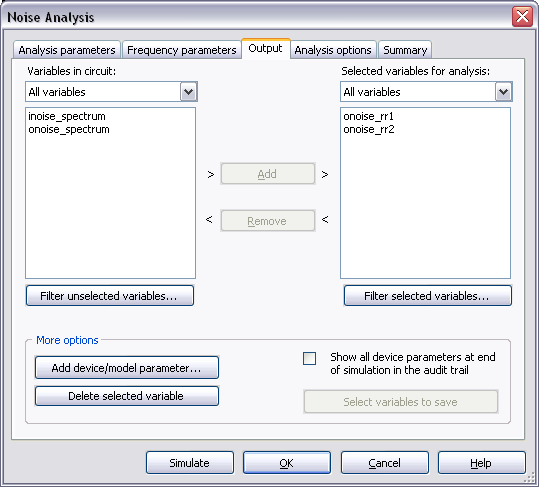Figure 6. Output variables for the Noise Analysis.

1. Click Simulate. The Grapher View shows the noise spectral density curves (Figure 7).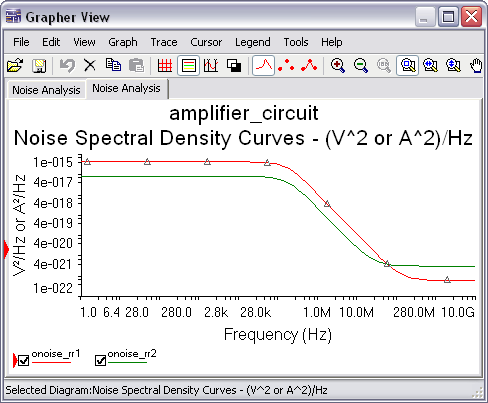Figure 7. Noise spectral density curves.

As you can see, the  graph shows that the noise voltage is constant for lower frequencies. For higher  frequencies the noise voltage drops considerably.

Entering Expressions in Analyses in Multisim

SPICE Analysis Fundamentals

Circuit Design Technical Library

Join the NI Circuit Design Community